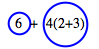### Home > MC1 > Chapter 3 > Lesson 3.1.1 > Problem3-7

3-7.

Copy each expression below and circle the terms. Then calculate the value of each expression.

1.  $6+4(2+3)$

It may help to review some of the knowledge you have about the Order of Operations.

This is what your circles should look like. Be sure to add up the sum and show your work!1.  $(6+4)(2+3)$

Because the addition signs are inside parentheses, we call this just one term. The parentheses tell us to simplify the sums before multiplying.

$50$

1.  $6+4·2+3$

First rewrite the expression. Now, it's time to draw the circles around any terms you see. Remember, these terms are separated by addition ($+$) symbols.

$6+4·2+3$

Now, can you simplify the terms and find the sum?